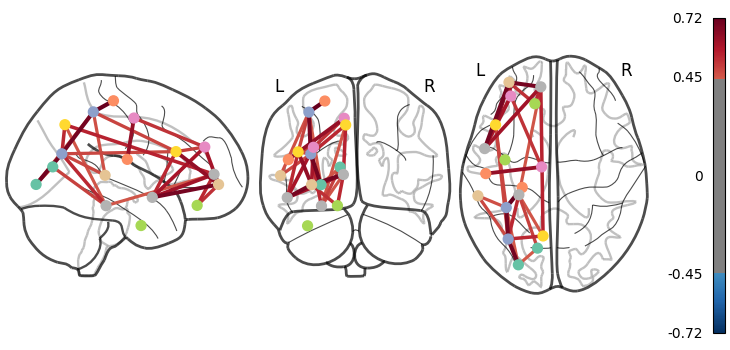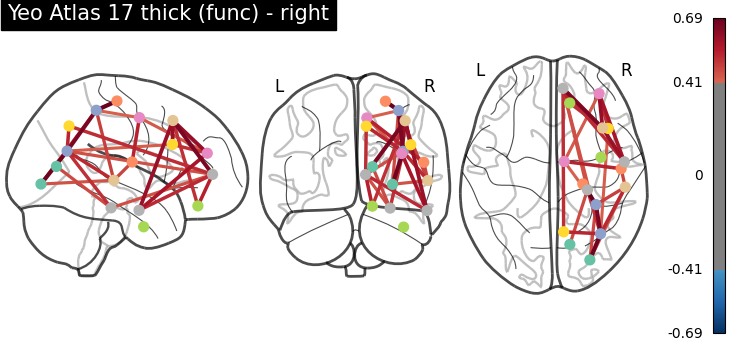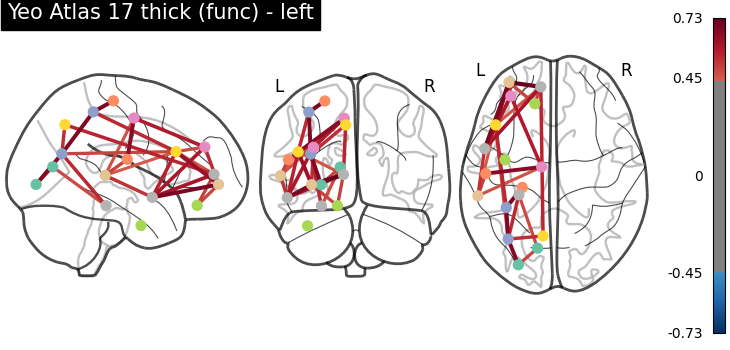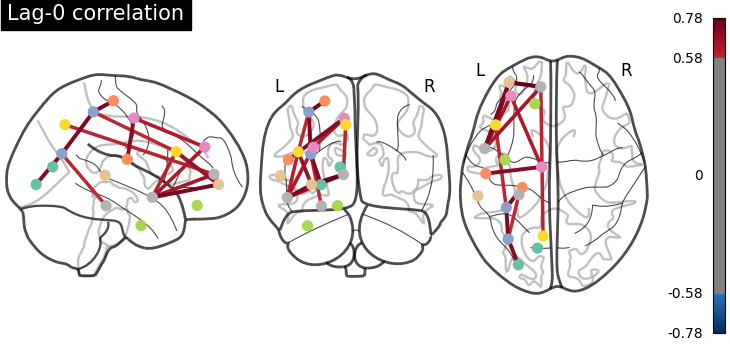# Comparing connectomes on different reference atlases#

This examples shows how to turn a parcellation into connectome for visualization. This requires choosing centers for each parcel or network, via `nilearn.plotting.find_parcellation_cut_coords` for parcellation based on labels and `nilearn.plotting.find_probabilistic_atlas_cut_coords` for parcellation based on probabilistic values.

In the intermediary steps, we make use of `nilearn.maskers.MultiNiftiLabelsMasker` and `nilearn.maskers.MultiNiftiMapsMasker` to extract time series from nifti objects from multiple subjects using different parcellation atlases.

The time series of all subjects of the brain development dataset are concatenated and given directly to `nilearn.connectome.ConnectivityMeasure` for computing parcel-wise correlation matrices for each atlas across all subjects.

Mean correlation matrix is displayed on glass brain on extracted coordinates.

Note

If you are using Nilearn with a version older than `0.9.0`, then you should either upgrade your version or import maskers from the `input_data` module instead of the `maskers` module.

That is, you should manually replace in the following example all occurrences of:

```from nilearn.maskers import NiftiMasker
```

with:

```from nilearn.input_data import NiftiMasker
```

```from nilearn import datasets

yeo = datasets.fetch_atlas_yeo_2011()
print(
"Yeo atlas nifti image (3D) with 17 parcels and liberal mask "
f" is located at: {yeo['thick_17']}"
)
```
```Yeo atlas nifti image (3D) with 17 parcels and liberal mask  is located at: /home/runner/work/nilearn/nilearn/nilearn_data/yeo_2011/Yeo_JNeurophysiol11_MNI152/Yeo2011_17Networks_MNI152_FreeSurferConformed1mm_LiberalMask.nii.gz
```

```data = datasets.fetch_development_fmri(n_subjects=10)

print(
"Functional nifti images (4D, e.g., one subject) "
f"are located at : {data.func!r}"
)
print(
"Counfound csv files (of same subject) are located "
f"at : {data['confounds']!r}"
)
```
```Functional nifti images (4D, e.g., one subject) are located at : '/home/runner/work/nilearn/nilearn/nilearn_data/development_fmri/development_fmri/sub-pixar123_task-pixar_space-MNI152NLin2009cAsym_desc-preproc_bold.nii.gz'
Counfound csv files (of same subject) are located at : '/home/runner/work/nilearn/nilearn/nilearn_data/development_fmri/development_fmri/sub-pixar123_task-pixar_desc-reducedConfounds_regressors.tsv'
```

## Extract coordinates on Yeo atlas - parcellations#

```from nilearn.connectome import ConnectivityMeasure

# ConenctivityMeasure from Nilearn uses simple 'correlation' to compute
# connectivity matrices for all subjects in a list
connectome_measure = ConnectivityMeasure(
kind="correlation",
standardize="zscore_sample",
)

# useful for plotting connectivity interactions on glass brain
from nilearn import plotting

# atlas parcels from multiple subjects using parallelization to speed up the
# computation
labels_img=yeo["thick_17"],
standardize="zscore_sample",
standardize_confounds="zscore_sample",
memory="nilearn_cache",
n_jobs=2,
)

# extract time series from all subjects

# calculate correlation matrices across subjects and display
correlation_matrices = connectome_measure.fit_transform(time_series)

# Mean correlation matrix across 10 subjects can be grabbed like this,
# using connectome measure object
mean_correlation_matrix = connectome_measure.mean_

# grab center coordinates for atlas labels
coordinates = plotting.find_parcellation_cut_coords(labels_img=yeo["thick_17"])

# plot connectome with 80% edge strength in the connectivity
plotting.plot_connectome(
mean_correlation_matrix,
coordinates,
edge_threshold="80%",
title="Yeo Atlas 17 thick (func)",
)
``````<nilearn.plotting.displays._projectors.OrthoProjector object at 0x7fb9b2585610>
```

## Plot a directed connectome - asymmetric connectivity measure#

In this section, we use the lag-1 correlation as the connectivity measure, which leads to an asymmetric connectivity matrix. The plot_connectome function accepts both symmetric and asymmetric matrices, but plot the latter as a directed graph.

```import numpy as np

# Define a custom function to compute lag correlation on the time series
def lag_correlation(time_series, lag):
n_subjects = len(time_series)
n_samples, n_features = time_series.shape
lag_cor = np.zeros((n_subjects, n_features, n_features))
for subject, serie in enumerate(time_series):
for i in range(n_features):
for j in range(n_features):
if lag == 0:
lag_cor[subject, i, j] = np.corrcoef(
serie[:, i], serie[:, j]
)[0, 1]
else:
lag_cor[subject, i, j] = np.corrcoef(
serie[lag:, i], serie[:-lag, j]
)[0, 1]
return np.mean(lag_cor, axis=0)

# Compute lag-0 and lag-1 correlations and plot associated connectomes
for lag in [0, 1]:
lag_correlation_matrix = lag_correlation(time_series, lag)
plotting.plot_connectome(
lag_correlation_matrix,
coordinates,
edge_threshold="90%",
title=f"Lag-{lag} correlation",
)
```
••```/usr/share/miniconda3/envs/testenv/lib/python3.9/site-packages/nilearn/plotting/displays/_projectors.py:190: UserWarning:

'adjacency_matrix' is not symmetric. A directed graph will be plotted.
```

## Load probabilistic atlases - extracting coordinates on brain maps#

```dim = 64
difumo = datasets.fetch_atlas_difumo(
dimension=dim, resolution_mm=2, legacy_format=False
)
```

## Iterate over fetched atlases to extract coordinates - probabilistic#

```from nilearn.maskers import MultiNiftiMapsMasker

# atlas parcels from multiple subjects using parallelization to speed up the
# # computation
maps_img=difumo.maps,
standardize="zscore_sample",
standardize_confounds="zscore_sample",
memory="nilearn_cache",
n_jobs=2,
)

# extract time series from all subjects

# calculate correlation matrices across subjects and display
correlation_matrices = connectome_measure.fit_transform(time_series)

# Mean correlation matrix across 10 subjects can be grabbed like this,
# using connectome measure object
mean_correlation_matrix = connectome_measure.mean_

# grab center coordinates for probabilistic atlas
coordinates = plotting.find_probabilistic_atlas_cut_coords(
maps_img=difumo.maps
)

# plot connectome with 85% edge strength in the connectivity
plotting.plot_connectome(
mean_correlation_matrix,
coordinates,
edge_threshold="85%",
title=f"DiFuMo with {dim} dimensions (probabilistic)",
)
plotting.show()
``````/usr/share/miniconda3/envs/testenv/lib/python3.9/site-packages/nilearn/_utils/cache_mixin.py:241: UserWarning:

memory_level is currently set to 0 but a Memory object has been provided. Setting memory_level to 1.
```

Total running time of the script: (2 minutes 33.597 seconds)

Estimated memory usage: 1727 MB

Gallery generated by Sphinx-Gallery